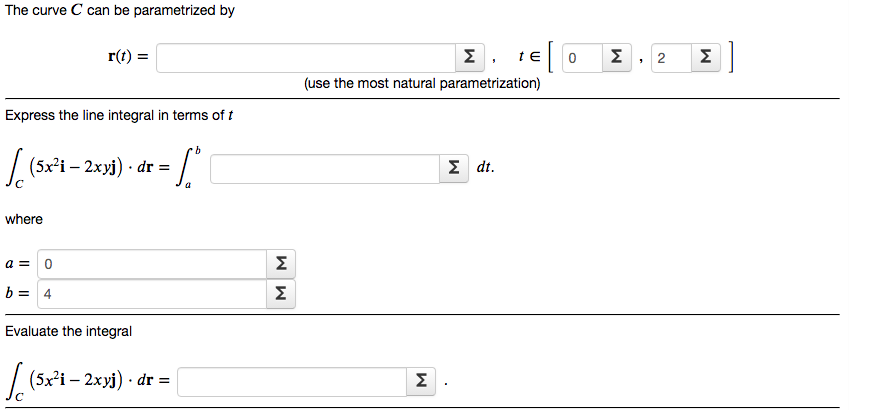# The curve C can be parametrized by r(t) = Σ , te Σ Σ | (use the most natural parametrization) Express the line integral in terms of t 9. / (5x-i – 2xyj) · dr = Σdt. where Σ 4 Σ Evaluate the integral | (5x²i – 2xyj) · dr = Σ 2.

Question

Find the work done by the force field F(x,y)=5x2i−2xyj on a particle that moves once around the circle x^2+y^2=16 oriented in the counter-clockwise direction.help_outlineImage TranscriptioncloseThe curve C can be parametrized by r(t) = Σ , te Σ Σ | (use the most natural parametrization) Express the line integral in terms of t 9. / (5x-i – 2xyj) · dr = Σdt. where Σ 4 Σ Evaluate the integral | (5x²i – 2xyj) · dr = Σ 2. fullscreen